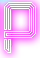# [Challenge] JawBreaker Game

#### Statement

The goal of this popular game is to get maximum number of points, by removing aligned pieces of the same color. Pieces can only be removed if they touch each other by an entire side. The more pieces you remove in one turn, the more points you get. The number of points in one turn is described by the following formula: N*(N-1), where N is the number of pieces (for example 2*(2-1)=2, 10*(10-1)=90). If you remove pieces from the middle of the field, then all pieces located higher fall down and occupy the empty spaces. The game is finished when no pieces which can be removed from field remain.In this problem you will be given a field and pieces on it. Your goal is to obtain the maximum number of points.

Note: You can practice a little and plan your strategy with this on-line game [the on-line game is slightly different from the one described above]: http://www.bigfrog.net/jawbreaker/

#### Input:

t – the number of tests, then t tests follow.

Each test starts with 3 integers: H - the number of rows of the playing field, W - the number of columns of the playing field and C - the number of different colors of pieces. Then follow H rows with W numbers in each, separated by spaces. Each number is in the range from 0 to C-1 and describes the color of a piece.

#### Output:

For each test you must output the letter “Y” if you want to solve this test, or the letter “N” otherwise. If you output Y, you must output a set of lines with 2 integers x, y in each. These integers define rows and columns in the field. [0 ≤ x < H], [0 ≤ y < W]. Coordinates are counted from the upper left corner of field. After your last move output the line -1 -1. You’ll receive status Wrong Answer if your coordinates are outside the field, or point to an empty space, or to a single piece.

#### Score

The score received for this problem is sum of points received for each playing field. The score for a playing field is calculated as ⌊(100 * C * C * base_score) / (H * W)⌋, where C – number of different colors, H – field height, W – field width, base_score – number of points calculated as in the description of problem.

#### Constraints:

• t ≤ 500
• 4 ≤ H, W ≤ 50
• 3 ≤ C ≤ 20

```1
4 4 3
0 0 1 1
1 1 2 2
0 1 2 0
0 1 1 2
```

```Y
1 0
1 0
3 2
-1 -1
```

• 4 seconds
• 256MB

#### Explanation:

Initial field:

```0 0 1 1
1 1 2 2
0 1 2 0
0 1 1 2
```

After the first turn (removed 5 "ones"):

```. . . 1
0 . 1 2
0 . 2 0
0 0 2 2
```

After the second turn (removed 4 "zeros"):

```. . . 1
. . 1 2
. . 2 0
. . 2 2
```

After the third turn (removed 3 "twos"):

```. . . .
. . . 1
. . . 2
. . 1 0
```

Score:

In this case base_score = 5*(5-1) + 4*(4-1) + 3*(3-1) = 20 + 12 + 6 = 38,
so score = ⌊(100*3*3*38)/(4*4)⌋ = ⌊2137.5⌋ = 2137.

Problem source: SPOJ